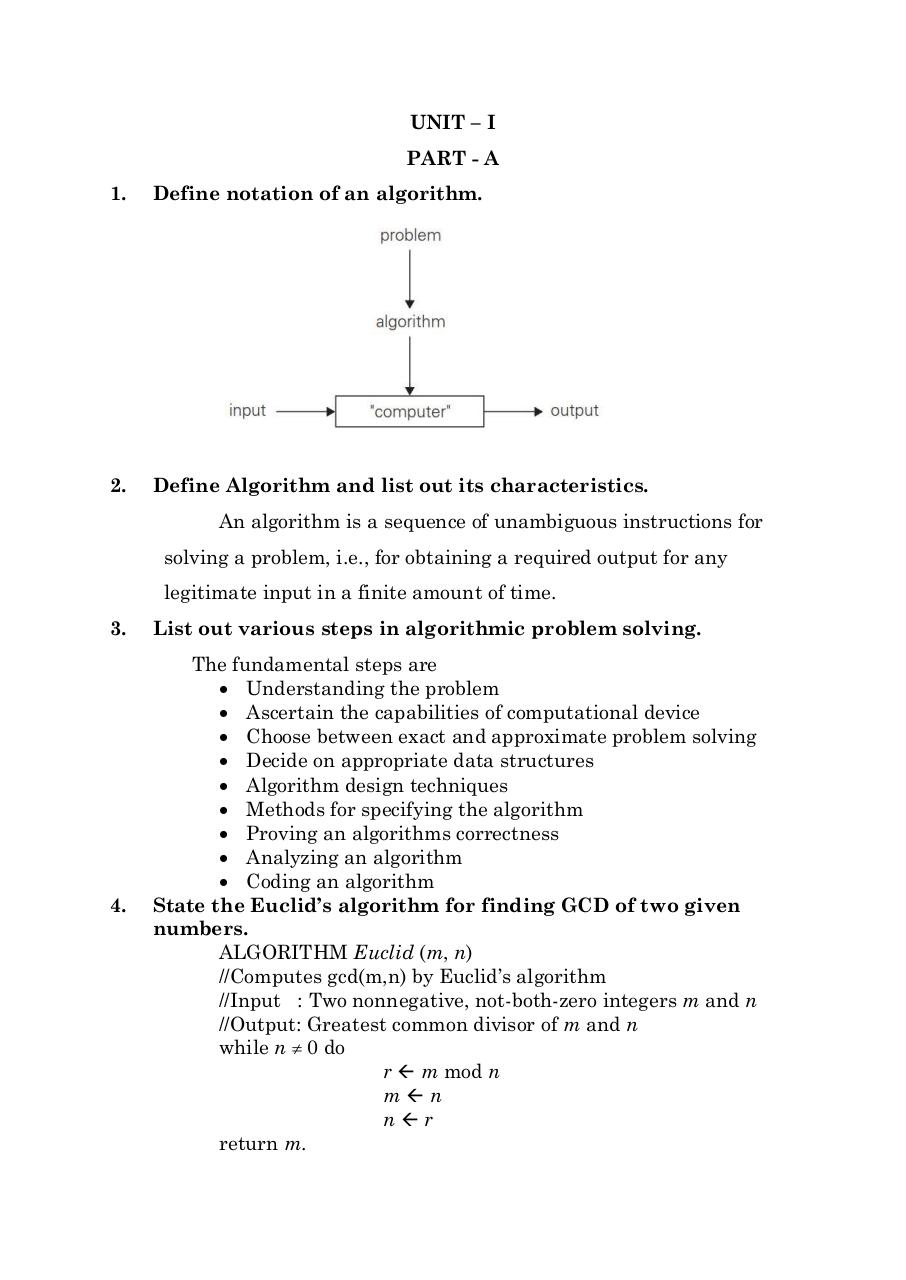# UNIT 1 QB.pdfPage 1 2 3 4 5 6 7 8

#### Text preview

UNIT – I
PART - A
1.

Define notation of an algorithm.

2.

Define Algorithm and list out its characteristics.
An algorithm is a sequence of unambiguous instructions for
solving a problem, i.e., for obtaining a required output for any
legitimate input in a finite amount of time.

3.

List out various steps in algorithmic problem solving.

4.

The fundamental steps are
 Understanding the problem
 Ascertain the capabilities of computational device
 Choose between exact and approximate problem solving
 Decide on appropriate data structures
 Algorithm design techniques
 Methods for specifying the algorithm
 Proving an algorithms correctness
 Analyzing an algorithm
 Coding an algorithm
State the Euclid’s algorithm for finding GCD of two given
numbers.
ALGORITHM Euclid (m, n)
//Computes gcd(m,n) by Euclid’s algorithm
//Input : Two nonnegative, not-both-zero integers m and n
//Output: Greatest common divisor of m and n
while n  0 do
r  m mod n
mn
nr
return m.